Next: Cold Emission Up: One-Dimensional Potentials Previous: Square Potential Barrier

# WKB Approximation

Consider a particle of massand energy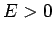moving through some slowly varying potential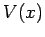. The particle's wavefunction satisfies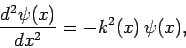(337)

where(338)

Let us try a solution to Eq. (337) of the form(339)

where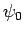is a complex constant. Note that this solution represents a particle propagating in the positive-direction [since the full wavefunction is multiplied by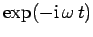, where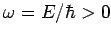] with the continuously varying wavenumber. It follows that(340)

and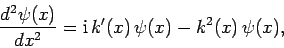(341)

where. A comparison of Eqs. (337) and (341) reveals that Eq. (339) represents an approximate solution to Eq. (337) provided that the first term on its right-hand side is negligible compared to the second. This yields the validity criterion, or(342)

In other words, the variation length-scale of, which is approximately the same as the variation length-scale of, must be much greater than the particle's de Broglie wavelength (which is of order). Let us suppose that this is the case. Incidentally, the approximation involved in dropping the first term on the right-hand side of Eq. (341) is generally known as the WKB approximation.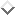Similarly, Eq. (339) is termed a WKB solution.

According to the WKB solution (339), the probability density remains constant: i.e.,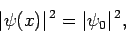(343)

as long as the particle moves through a region in which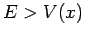, andis consequently real (i.e., an allowed region according to classical physics). Suppose, however, that the particle encounters a potential barrier (i.e., a region from which the particle is excluded according to classical physics). By definition,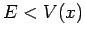inside such a barrier, andis consequently imaginary. Let the barrier extend from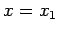to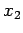, where. The WKB solution inside the barrier is written(344)

where(345)

Here, we have neglected the unphysical exponentially growing solution.

According to the WKB solution (344), the probability density decays exponentially inside the barrier: i.e.,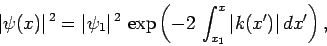(346)

whereis the probability density at the left-hand side of the barrier (i.e.,). It follows that the probability density at the right-hand side of the barrier (i.e.,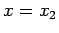) is(347)

Note that. Of course, in the region to the right of the barrier (i.e.,), the probability density takes the constant value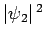.

We can interpret the ratio of the probability densities to the right and to the left of the potential barrier as the probability,, that a particle incident from the left will tunnel through the barrier and emerge on the other side: i.e.,(348)

(see Sect. 5.3). It is easily demonstrated that the probability of a particle incident from the right tunneling through the barrier is the same.

Note that the criterion (342) for the validity of the WKB approximation implies that the above transmission probability is very small. Hence, the WKB approximation only applies to situations in which there is very little chance of a particle tunneling through the potential barrier in question. Unfortunately, the validity criterion (342) breaks down completely at the edges of the barrier (i.e., atand), since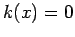at these points. However, it can be demonstrated that the contribution of those regions, aroundand, in which the WKB approximation breaks down to the integral in Eq. (348) is fairly negligible. Hence, the above expression for the tunneling probability is a reasonable approximation provided that the incident particle's de Broglie wavelength is much smaller than the spatial extent of the potential barrier.Next: Cold Emission Up: One-Dimensional Potentials Previous: Square Potential Barrier
Richard Fitzpatrick 2010-07-20